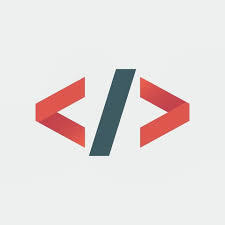# Datatypes in R language

For Programming in any language, variables play an important role. Variables are used to reserve the space in memory and then we can utilize it by storing some value in that variable.
The value stored can be of any type. For example, integer, float, double, string, or boolean. All these data types are used to reserve different spaces in memory.
Unlike other programming languages, data types of R language are not same as them. In R language the variables are assigned with R objects and the datatype of R Objects becomes the data type of variable.
Data types in R language are:

• Vectors
• List
• Matrices
• Arrays
• Factors
• Data Frames

The easiest and simplest of these is Vectors. There are 6 subdivisions of the vectors and they are known as atomic vectors, also known as 6 classes of vectors.

#### Classes of Vectors

Data Types Example Verify
Logical True, False

 `v<``-``False` `print``(``class``(v)) `

Output:

`"logical"`
Numeric 11, 5.6, 0.99

 `v<``-``2.3` `print``(``class``(v)) `

Output:

`"numeric"`
Integer 3L, 6L, 10L

 `v<``-``4L` `print``(``class``(v)) `

Output:

`"integer"`
Complex 6+i

 `v<``-``3` `+` `4i` `print``(``class``(v)) `

Output:

`"complex"`
Character ‘R’, “Language”

 `v<``-``"Geeks"` `print``(``class``(v)) `

Output:

`"character"`
Raw “Hello” is stored as 48 65 6c 6c 6f

 `v<``-``charToRaw``"Hello"` `print``(``class``(v)) `

Output:

`"raw"`

Please remember that in the R language the number of classes is not limited to the above types. Utilizing the atomic vectors we can create an array whose class can be further considered to be an array.

#### Vectors

`c()` function is used to combine elements when more than one element is to be used in vector.

 `# Create a vector. ` `apple <``-` `c(``'geeks'``, ``'for'``, ``"benefit"``) ` `print``(geeks) ` ` `  `# Get the class of the vector. ` `print``(``class``(geeks)) `

Output:

```"geeks" "for" "benefit"
"character"```

#### List

The list is a kind of R object which can combine more than one element even another list inside it.

 `# Create a list. ` `list1 <``-` `list``(c(``4``, ``5``, ``6``), ``22.7``, cos) ` ` `  `# Print the list. ` `print``(list1) `

Output:

```[]
 4, 5, 6

[]
 22.7

[]
function (x)  .Primitive("cos")```

#### Matrices

It is a data set having two-dimension. It can be created using the vector input with the use of `matrix()` function.

 `# Create a matrix. ` `S ``=` `matrix(c(``'a'``, ``'b'``, ``'c'``, ``'d'``, ``'e'``, ``'f'``),  ` `           ``numberOfRow ``=` `2``, numberOfCol ``=` `3``,  ` `           ``byRow ``=` `TRUE) ` `print``(M) `

Output:

```    [, 1] [, 2] [, 3]
[1, ] "a"  "b"  "c"
[2, ] "d"  "e"  "f"```

#### Arrays

Unlike matrices, arrays can be of multiple dimensions. In array “dim” variables takes the number of dimensions we need to create.

 `# Create an array. ` `a <``-` `array(c(``'grapes'``, ``'mango'``), dim ``=` `c(``3``, ``3``, ``2``)) ` `print``(a) `

Output:

```,, 1

[, 1]       [, 2]     [, 3]
[1, ] "grapes" "mango"   "grapes"
[2, ] "mango"  "grapes"  "mango"
[3, ] "grapes" "mango"   "grapes",, 2

[, 1]       [, 2]      [, 3]
[1, ] "mango"  "grapes"  "mango"
[2, ] "grapes" "mango"   "grapes"
[3, ] "mango"  "grapes"  "mango" ```

#### Factors

Vectors are used to create factors that are R-Objects. Along with the distinct value of elements factors store vectors as labels.
Labels are always a character. `factor()` function is used to create factors. `nlevel()` function always counts the number of levels.

 `# Create a vector ` `colors <``-` `c(``'green'``, ``'green'``, ``'yellow'``,  ` `            ``'red'``, ``'red'``, ``'red'``, ``'green'``) ` ` `  `# Create a factor object. ` `factor <``-` `factor(colors) ` ` `  `# Print the factor. ` `print``(factor) ` `print``(nlevels(factor)) `

Output:

``` green  green  yellow red    red    red    green
Levels: green red yellow
 3```

#### Data Frames

Tabular data R-Objects are known as data frames. Basically it is kind of the matrix. But unlike matrix, data frames can store different forms of modes. It is a vector having the same length. `data.frames()` function is used to create data frames.

 `# Create the data frame. ` `employee <``-` `data.frame( ` `   ``gender ``=` `c(``"Male"``, ``"Male"``, ``"Female"``),  ` `   ``height ``=` `c(``152``, ``171.5``, ``165``),  ` `   ``weight ``=` `c(``81``, ``93``, ``78``), ` `   ``Age ``=` `c(``42``, ``38``, ``26``) ` `) ` `print``(employee) `

Output:

```gender height weight Age
1   Male  152.0     81  42
2   Male  171.5     93  38
3 Female  165.0     78  26  ```

My Personal Notes arrow_drop_upCheck out this Author's contributed articles.

If you like GeeksforGeeks and would like to contribute, you can also write an article using contribute.geeksforgeeks.org or mail your article to contribute@geeksforgeeks.org. See your article appearing on the GeeksforGeeks main page and help other Geeks.

Please Improve this article if you find anything incorrect by clicking on the "Improve Article" button below.

Article Tags :

Be the First to upvote.

Please write to us at contribute@geeksforgeeks.org to report any issue with the above content.